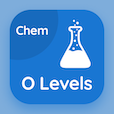Cambridge Online Courses (GCSE)

O Level Chemistry MCQs

O Level Chemistry MCQ PDF - Topics

# Relative Molecular Mass MCQ Quiz Online

Learn Relative Molecular Mass Multiple Choice Questions (MCQ), Relative Molecular Mass quiz answers PDF to study o level chemistry online course for o level chemistry classes. Chemical Formulae and Equations Multiple Choice Questions and Answers (MCQs), Relative Molecular Mass quiz questions for SAT practice test. "Relative Molecular Mass MCQ" PDF Book: valency and chemical formula, chemical symbols test prep for two year degree programs.

"Molecular weight of propane (C3H8) is" MCQ PDF: relative molecular mass with choices 44.095 g/mol, 40.01 g/mol, 41.01 g/mol, and 46.02 g/mol for SAT practice test. Study relative molecular mass quiz questions for merit scholarship test and certificate programs for online colleges for teaching.

## MCQs on Relative Molecular Mass Quiz

MCQ: Molecular weight of propane (C3H8) is

44.095 g/mol
40.01 g/mol
41.01 g/mol
46.02 g/mol

MCQ: Relative molecular mass of Na2CO3 is

98
128
286
198

MCQ: Relative formula mass of aluminum sulphate (NH4)2SO4 is

132.14 g/mol
113.14 g/mol
139.33 g/mol
143.69 g/mol

MCQ: The greatest proportion by mass of nitrogen is in

Hydrazine (N2H4),
ammonium nitrate (NH4NO3)
ammonium sulphate (NH4)2SO4
urea (NH2CONH2)

MCQ: Relative molecular masses can be calculated by the

relative atomic mass
relative mass of a covalent compound
relative atomic masses of individual atoms

### More Topics from O Level Chemistry Course